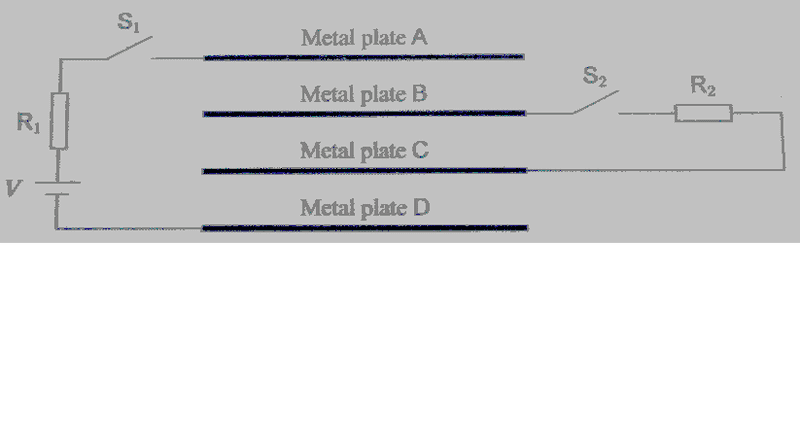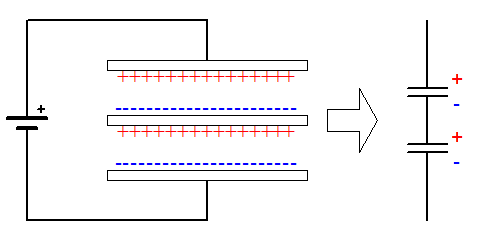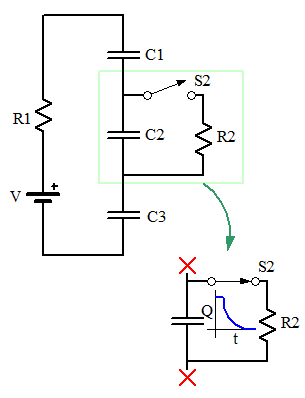# Charge on Parallel Plates

## Homework Statement

Four uncharged metal plates of the same area are placed in vacuum and separated by equal distances. They are connected by conducting wires to two resistors, two open switches and a battery. The thickness of the plates is negligible, and their area is sufficiently large. S1 is now closed, and after sufficient time elapses, a charge with quantity Q is stored in A. Next, as S1 is kept closed, S2 is also closed. After sufficient time elapses, a charge with quantity of electricity Q' is stored in A. What is the ratio of Q'/Q?Q = C.V
C = ε0 . A / d

## The Attempt at a Solution

I think the circuit acts as capacitor.

When S1 is closed and S2 is open, the circuit is like capacitor between plate A and D because potential difference only applied to the two plates by the battery. Assume the distance between two adjacent plates is d, then the distance of AD = 3d. So the charge stored on A = ε0 . A / 3d . V

When both switches closed, I don't have idea how the circuit works....

## Answers and Replies

gneill
Mentor
Here's a hint for these types of problems: When plates are stacked in parallel like this, adjacent pairs behave as individual capacitors, and these capacitors are effectively connected in series.

•songoku
Here's a hint for these types of problems: When plates are stacked in parallel like this, adjacent pairs behave as individual capacitors, and these capacitors are effectively connected in series.

1. How can adjacent pairs behave as individual capacitors?
2. When both switches closed, is it still capacitor?

gneill
Mentor
1. How can adjacent pairs behave as individual capacitors?
2. When both switches closed, is it still capacitor?

1. The electric field induces charge separation on intervening plates. The facing plate surfaces act as capacitors.2. Yes. But you should draw the equivalent circuit to see what closing S2 does to the circuit. Note that the values of the resistors are unimportant as you're looking for steady-state conditions.

•songoku
1. The electric field induces charge separation on intervening plates. The facing plate surfaces act as capacitors.
View attachment 105514

2. Yes. But you should draw the equivalent circuit to see what closing S2 does to the circuit. Note that the values of the resistors are unimportant as you're looking for steady-state conditions.

Sorry for late reply

When both switches are closed, positive charge will accumulate on plate A and negative charge will accumulate on plate D. I am not sure how to proceed then.

Plate A will induce negative charge on plate B and plate D will induce positive charge on plate C. Although S2 is closed, there won't be any change on charge accumulated on plate B and C because it is not closed circuit??

gneill
Mentor
It might be easier to see what is going on if you draw the circuit diagram that is equivalent to the plate setup. Each gap between plates represents a capacitor and they are all connected in series. So before switch two closes there are three capacitors of equal value in series with the battery V and resistor R. At steady state what potential do you expect to see across each of the capacitors, and hence what's an expression for their charges?

•songoku
I can imagine there will be three capacitors connected in series when switch 2 is open. If we assume the capacitance of each capacitor is C, the total capacitance will be C/3 and the value of Q will be C/3 . V

I don't understand what will happen to the circuit when switch 2 is closed. Because plate B and C are now connected by wire, the circuit will consist only two capacitors connected in series (capacitor AB and capacitor CD)?

gneill
Mentor
I can imagine there will be three capacitors connected in series when switch 2 is open. If we assume the capacitance of each capacitor is C, the total capacitance will be C/3 and the value of Q will be C/3 . V
Yes, good.
I don't understand what will happen to the circuit when switch 2 is closed. Because plate B and C are now connected by wire, the circuit will consist only two capacitors connected in series (capacitor AB and capacitor CD)?
A sufficient time after the switch S2 closes the circuit will reach steady state, and because of the capacitors in the path to the voltage source that means no current flowing (Except for transient activity capacitors block DC current).

Now, the center capacitor has a resistor across it via the closed switch. What does that tell you about the steady state potential and charge on that capacitor?•songoku
A sufficient time after the switch S2 closes the circuit will reach steady state, and because of the capacitors in the path to the voltage source that means no current flowing (Except for transient activity capacitors block DC current).

Now, the center capacitor has a resistor across it via the closed switch. What does that tell you about the steady state potential and charge on that capacitor?
View attachment 106406

Let me try.

The energy stored in the center capacitor will be used up so there is no charge accumulated on the plates of C2. C2 will behave like short circuit so now the circuit consists only two capacitors (C1 and C3) in series?

gneill
Mentor
Let me try.

The energy stored in the center capacitor will be used up so there is no charge accumulated on the plates of C2. C2 will behave like short circuit so now the circuit consists only two capacitors (C1 and C3) in series?
Well, I'd say that at steady state the potential across C2 will be zero. But essentially you are correct in that C1 and C3 will be the only capacitors holding a charge. So what must be the charge on them?

•songoku
Well, I'd say that at steady state the potential across C2 will be zero. But essentially you are correct in that C1 and C3 will be the only capacitors holding a charge. So what must be the charge on them?

The total capacitance is C/2, so the value of Q' = C/2 . V and the ratio of Q'/Q will be 3/2

gneill
Mentor
Yes, that looks right.

Yes, that looks right.

Thank you very much